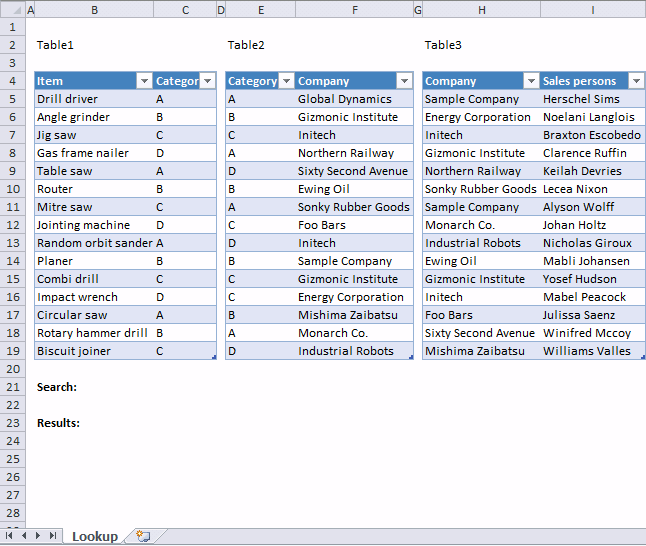Author: Oscar Cronquist Article last updated on January 14, 2018

It can be really hard trying to follow a lookup in related tables. I will show you how to use conditional formatting for easy identification.If you add more rows to the tables, the conditional formatting expands automatically.

Conditional formatting formula applied to table1:

=INDIRECT("Table1[@Item]")=\$C\$21

Conditional formatting formula applied to table2:

=INDEX(INDIRECT("Table1[Category]"), MATCH(\$C\$21, INDIRECT("Table1[Item]"), 0))=INDIRECT("Table2[@Category]")

Conditional formatting formula applied to table3:

=AND(ISERROR(MATCH(INDIRECT("Table3[@Company]"), IF(ISERROR(MATCH(INDIRECT("Table2[Category]"), IF(\$C\$21=INDIRECT("Table1[Item]"), INDIRECT("Table1[Category]"), ""), 0)), "", INDIRECT("Table2[Company]")), 0)))=FALSE

You can find the array formula in cell C23 here:

Lookups in three related tables and return multiple values